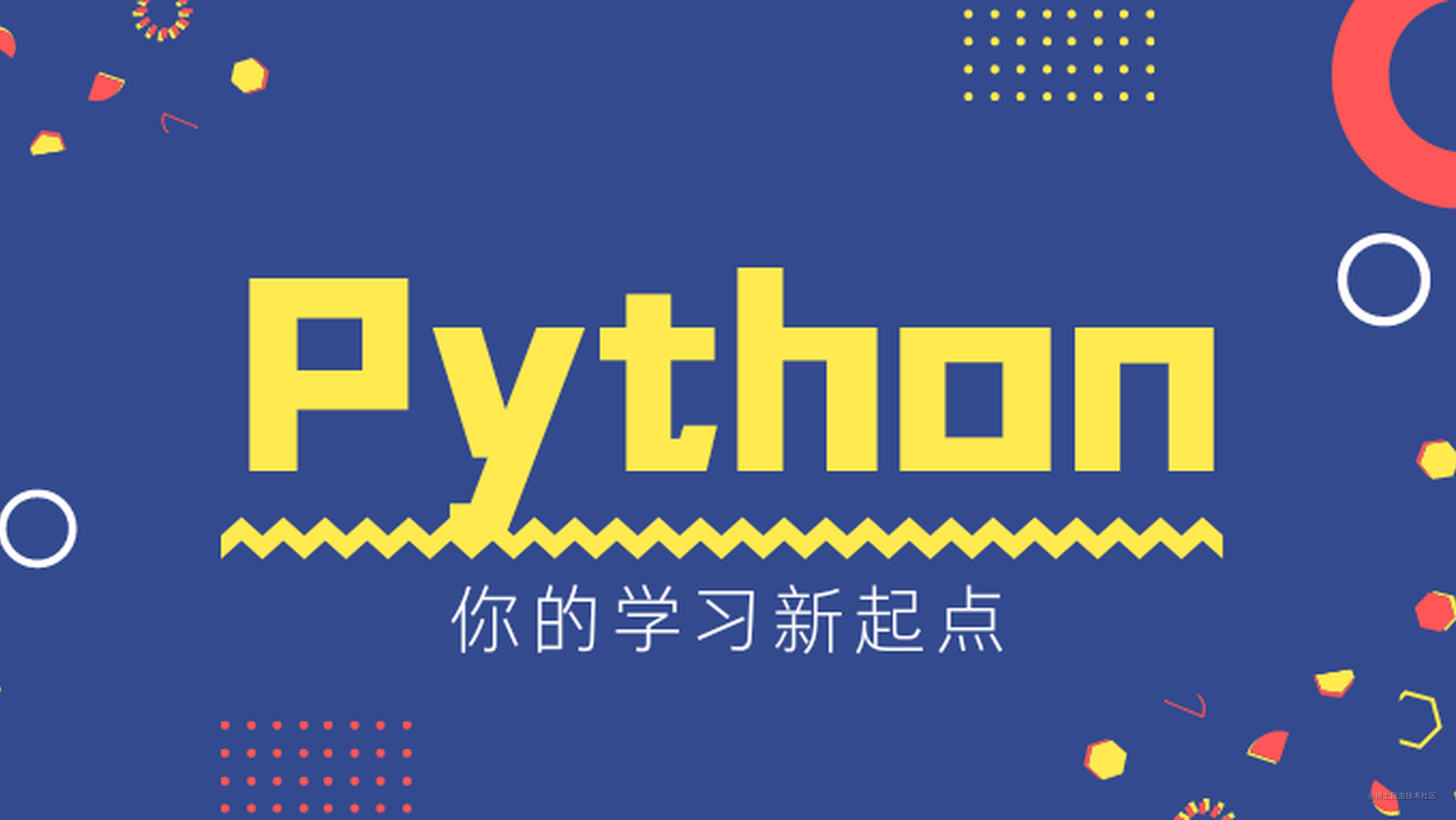# 【Python基础】详解Python基础函数，包教包会（二）「这是我参与11月更文挑战的第5天，活动详情查看：2021最后一次更文挑战」。

## 运行环境

python：3.8.3
jupyter-notebook : 6.4.0

## 列表操作

### list()

`list()` 将可迭代对象转成列表。

``````list((0,1,2)) + list({0,1,2}) + list('012')

output：[0, 1, 2, 0, 1, 2, '0', '1', '2']

``````list(range(3))

output：[0, 1, 2]

### list.append()

``````lst = ['Python', 'Java']
lst.append('C')
lst

output：['Python', 'Java', 'C']

### list.extend()

`extend()` 在列表的末尾添加可迭代对象(列表、元组、字典、字符串)中的元素来扩展列表。

1️⃣ 追加列表

``````lst = ['Python', 'Java', 'C']
lst.extend([1, 2, 3])
lst

output：['Python', 'Java', 'C', 1, 2, 3]

2️⃣ 追加字符串

``````lst = ['Python', 'Java', 'C']
lst.extend('123')
lst

output：['Python', 'Java', 'C', '1', '2', '3']

3️⃣ 追加集合

``````lst = ['Python', 'Java', 'C']
lst.extend({1,2,3})
lst

output：['Python', 'Java', 'C', 1, 2, 3]

4️⃣ 追加字典

``````lst = ['Python', 'Java', 'C']
lst.extend({1: 'b', 2: 'a'})
lst

output：['Python', 'Java', 'C', 1, 2]

### list.insert()

`insert(index, object)` 将指定对象插入到列表的 `index` 位置，原本 `index` 位置上及 `> index` 的元素的元素整体后移。

``````lst = ['Python', 'Java', 'C']
lst.insert(1, 'C++')
lst

output：['Python', 'C++', 'Java', 'C']

``````lst = ['Python', 'Java', 'C']
lst.insert(6, 'C++')
lst

output：['Python', 'Java', 'C', 'C++']
`index` 的值大于列表长度时，会在列表末尾添加。

### list.pop()

`pop(index=-1)` 移除列表中指定位置元素(默认最后一个)，并且返回移除元素的值。若指定的 `index` 值超过列表长度则会报错。

``````lst = ['Python', 'Java', 'C']
lst.pop(1), lst

output：('Java', ['Python', 'C'])

### list.remove(元素)

`lst.remove(value)` 移除列表中第一次出现的 `value` ，无返回值，直接修改列表；如果 `value` 不在列表中则报错。

``````lst = ['Python', 'Java', 'C', 'Python']
lst.remove('Python')
lst

output：['Java', 'C', 'Python']

``````lst = ['Python', 'Java', 'C', 'Python']
lst.remove('HTML')
lst

output：ValueError: list.remove(x): x not in list
`HTML` 不在列表中，发生错误

### list.clear()

`list.clear()` 移除列表中所有的元素，无返回值。

``````lst = ['Python', 'Java', 'C']
lst.clear()
lst

output：[]

### list.index()

`index(value, start, stop)` 返回列表中查找的第一个与value匹配的元素下标，可通过 `[start, stop)` 指定查找范围。

``````lst = ['Python', 'Java', 'C',
'Python', 'Python']
lst.index('Python')

output：0

``````lst = ['Python', 'Java', 'C',
'Python', 'Python']
lst.index('Python', 1, 3)

output：ValueError: 'Python' is not in list

### list.count()

`count(value)` 返回 `value` 在列表中出现的次数。若未在列表中找到 `value` 则返回 0 。

``````lst = ['Python', 'Java', 'C',
'Python', 'Python']
lst.count('Python')

output：3

``````lst = ['Python', 'Java', 'C',
'Python', 'Python']
lst.count('Py')

output：0

### list.reverse()

`reverse()` 将列表逆序排列，无返回值。

``````lst = [1, 5, 9, 2]
lst.reverse()
lst

output：[2, 9, 5, 1]

### list.sort()

`sort()` 对列表进行指定方式的排序，修改原列表，该排序是稳定的(即两个相等元素的顺序不会因为排序而改变)。

• key： 指定可迭代对象中的每一个元素来按照该函数进行排序
• reverse： `False` 为升序，`True` 为降序。

``````lst = [1, 5, 9, 2]
lst.sort()
lst

output：[1, 2, 5, 9]

``````lst = ['Python', 'C', 'Java']
lst.sort(key=lambda x:len(x), reverse=False)
lst

output：['C', 'Java', 'Python']

### list.copy()

`copy()` 对列表进行拷贝，返回新生成的列表，这里的拷贝是浅拷贝，下面会说明为什么特意说它是浅拷贝

``````lst = [[1,2,3], 'a' ,'b']
lst_copy = lst.copy()
lst_copy.pop()
lst, lst_copy

output：([[1, 2, 3], 'a', 'b'], [[1, 2, 3], 'a'])
`lst` 进行 `copy` ，然后删除列表 `lst_copy` 的最后一个元素，此时的 `lst` 的最后一个元素并未被删除，说明两个列表指向的地址确实不一样。

``````lst = [[1,2,3], 'a' ,'b']
lst_copy = lst.copy()
lst_copy.pop()
lst_copy.pop()
lst, lst_copy

output：([[1, 2], 'a', 'b'], [[1, 2], 'a', 'b'])

### 扩展：直接赋值、浅拷贝、深拷贝

（1）直接赋值，传递对象的引用而已。原始列表改变，被赋值的对象也会做相同改变。

（2）浅拷贝，没有拷贝子对象，所以原始数据子对象改变，拷贝的子对象也会发生变化。

（3）深拷贝，包含对象里面的子对象的拷贝，所以原始对象的改变不会造成深拷贝里任何子元素的改变，二者完全独立。

1️⃣ 先看直接赋值

``````lst = [1,2,3,[1,2]]
list1 = lst

# --直接赋值--
lst.append('a')
list1.append('b')

print(lst,'地址:',id(lst))
print(list1,'地址:',id(list1))
# [1, 2, 3, [1, 2, 'b'], 'a'] 地址: 2112498512768
# [1, 2, 3, [1, 2, 'b'], 'a'] 地址: 2112498512768

2️⃣ 浅拷贝需要用到 `copy` 模块，或者用 `list.copy()` 效果一样

``````from copy import copy

lst = [1, 2, 3, [1, 2]]
list2 = copy(lst)

# --浅拷贝--
lst.append('a')
list2.append('b')

print(lst,'地址:',id(lst))
print(list2,'地址:',id(list2))
# [1, 2, 3, [1, 2, 'b'], 'a'] 地址: 2112501949184
# [1, 2, 3, [1, 2, 'b']] 地址: 2112495897728

`lst``list2` 地址不同，但子列表的地址仍是相同的，修改子列表中的元素时，二者都会受到影响。

3️⃣ 深拷贝需要用到 `copy` 模块中的 `deepcopy` ，此时两个列表完全独立。

``````from copy import deepcopy

lst = [1, 2, 3, [1, 2]]
list3 = deepcopy(lst)

# --深拷贝--
lst.append('a')
list3.append('b')

print(lst,'地址:',id(lst))
print(list3,'地址:',id(list3))
# [1, 2, 3, [1, 2], 'a'] 地址: 2112506144192
# [1, 2, 3, [1, 2, 'b']] 地址: 2112499460224